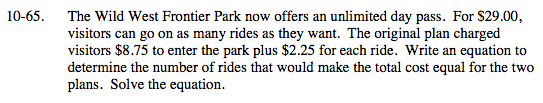### Home > MC2 > Chapter 10 > Lesson 10.1.5 > Problem10-65

10-65.Create a variable expression to represent this problem.
Use the number of rides as a variable.

Set the two parts of the equation equal to each other to find the value of the variable, or the number of rides, where both plans would have equal costs.

Consider $8.75 as a constant and that each ride costs$2.25 when writing your equation.

29 = 8.75 + 2.25x
x = 9 rides